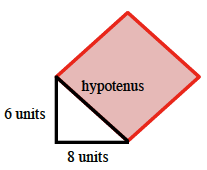Home > CCG > Chapter 2 > Lesson 2.3.1 > Problem2-104

2-104.

Draw a right triangle with legs of length $6$ and $8$ units, respectively, onto graph paper. Construct a square on the hypotenuse and use the square’s area to find the length of the hypotenuse. Homework Help ✎

Draw the triangle and a square where one side is the hypotenuse.Count the number of unit squares contained within the square, giving each partial square a value of one half.

Since the area of the square ($100$) is the length of the hypotenuse squared, the square root of the square's area is the length of the hypotenuse.

$\sqrt {100}=10$